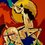# What's so special about 2014?Hello Everybody,

Now half of the year has passed, and most of us (including me) will be sitting for various Maths Olympiads, taking place in the next half all around the globe. For me, its NMTC and RMO.

The point is- These Olympiads are, as we all are aware, going to use the number 2014 in most of their questions. Thus, it will be beneficial for us to know, beforehand, some of the special properties of 2014 and the questions which can be asked. I searched the net for some eccentricities of 2014, and I wasn't able to find one.

Thus, I'd like you to comment any peculiarities of the number 2014 which you know, and, of course, try to post all questions you know related to 2014 with a #2014 tag.Note by Satvik Golechha
7 years, 1 month ago

This discussion board is a place to discuss our Daily Challenges and the math and science related to those challenges. Explanations are more than just a solution — they should explain the steps and thinking strategies that you used to obtain the solution. Comments should further the discussion of math and science.

When posting on Brilliant:

• Use the emojis to react to an explanation, whether you're congratulating a job well done , or just really confused .
• Ask specific questions about the challenge or the steps in somebody's explanation. Well-posed questions can add a lot to the discussion, but posting "I don't understand!" doesn't help anyone.
• Try to contribute something new to the discussion, whether it is an extension, generalization or other idea related to the challenge.

MarkdownAppears as
*italics* or _italics_ italics
**bold** or __bold__ bold
- bulleted- list
• bulleted
• list
1. numbered2. list
1. numbered
2. list
Note: you must add a full line of space before and after lists for them to show up correctly
paragraph 1paragraph 2

paragraph 1

paragraph 2

[example link](https://brilliant.org)example link
> This is a quote
This is a quote
    # I indented these lines
# 4 spaces, and now they show
# up as a code block.

print "hello world"
# I indented these lines
# 4 spaces, and now they show
# up as a code block.

print "hello world"
MathAppears as
Remember to wrap math in $$ ... $$ or $ ... $ to ensure proper formatting.
2 \times 3 $2 \times 3$
2^{34} $2^{34}$
a_{i-1} $a_{i-1}$
\frac{2}{3} $\frac{2}{3}$
\sqrt{2} $\sqrt{2}$
\sum_{i=1}^3 $\sum_{i=1}^3$
\sin \theta $\sin \theta$
\boxed{123} $\boxed{123}$

Sort by:

$2014=2\cdot 19\cdot 53$. This can save you a lot of time.

- 7 years, 1 month ago

The sum of the "non-obvious" prime factors of $2014$ (prime factors you can't really tell just by looking at the number, which here are $19$ and $53$) is the same as the sum of the non-obvious factors of $2013$ (which are $11$ and $61$).

I think I remember this fact being used on the AIME.

- 7 years, 1 month ago

Ahem! AFAIK, NMTC doesn't use any of the eccentric properties of any number. All they do is - employ this number in some form or the other in their questions! An example could be- They could describe a polynomial an ask you to find the $f(2014)$. But, I'm sure they wouldn't ask such easy questions though ;)

- 7 years, 1 month ago

Yes, but if they ask the sum of divisors of 2014, or something.....My intention was to collect as many questions about 2014 as I can......

- 7 years, 1 month ago

As I said before, "Questions would not be that easy". I'm sure, you know how to do it in a short way..Don't you? I will definitely help you in this endeavour though.

- 7 years, 1 month ago

Yes, and again, my intention is to collect questions on 2014.....

- 7 years, 1 month ago